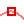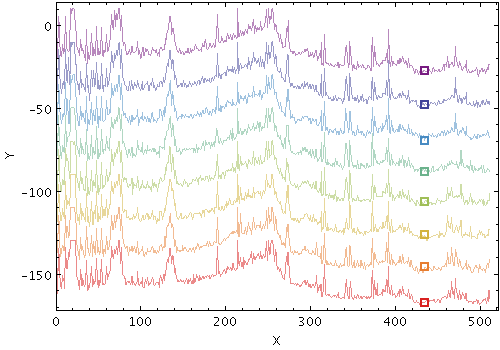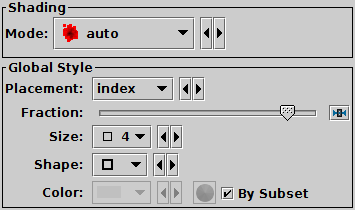Next Previous Up Contents
Next: YErrors Form
Up: Plot Forms
Previous: Marks Form

#### A.4.5.35 Handles Form

The Handles form (), available from the XYArray Layer Control, draws a single pointer (a "handle") somewhere near each group of points defined by pair of X/Y array coordinates. This doesn't do much to show the shape of the line formed by the array values, but it does provide a reference point for each line. This can be used by the parts of TOPCAT that associated a fixed position with each table row, in particular:

• you can click on the handle to activate the row it relates to, for instance to highlight the table row in the Data Window
• if the row is activated by some other action, its handle will be highlighted with the activation cursor
• you can define subsets graphically using the reference positions defined by the handles
Since array coordinates don't normally have a single per-row position, adding a Handles layer like this is the only way to perform those position-related activities with the plots from the XYArray Layer Control. Because of its general usefulness, a deactivated Handles control is added automatically to the layer control, so you just need to click the checkbox to display handles in this way.Example Handles plotHandles form configuration panel

The configuration options are:

See Appendix A.4.6.
Placement
Specifies where to draw the handle marker in relation to the X/Y array values. The options are:
• `index`: (X,Y) position at a certain fraction of the way through the arrays, as given by the Fraction value; Fraction=0.0 is the first element, Fraction=1.0 is the last
• `ymax`: (X,Y) position at which the maximum Y value is located (Fraction is ignored)
• `ymin`: (X,Y) position at which the minimum Y value is located (Fraction is ignored)
• `xmax`: (X,Y) position at which the maximum X value is located (Fraction is ignored)
• `xmin`: (X,Y) position at which the minimum X value is located (Fraction is ignored)
• `xymean`: center of gravity of all the (X,Y) points (Fraction is ignored)
Fraction
Provides a numeric value in the range 0..1 that may influence where the handle is placed. Currently, this is only relevant for a Placement value of index, where it indicates how far through the array the reference (X,Y) position should be taken (0.0 means the first element, 1.0 means the last). For other values of placement it is ignored.
Size
Marker size in pixels.
Shape
Marker shape from a list of options.

Next Previous Up Contents
Next: YErrors Form
Up: Plot Forms
Previous: Marks Form

TOPCAT - Tool for OPerations on Catalogues And Tables